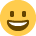# Gross Margin (quick question)

Hi all... dumb question and yes i know its late but .......

Cost of production equals (Say) £1500.... the question is...

If the target gross margin with this cost base is 48%, how much should we estimate for the job to the customer excl. Vat?

i am thinking 1500 * 48% but just dont look right this time of night?

Cheers

• Experienced Mentor Registered Posts: 591
I always find Margin and Mark up really tricky. Right lets see how I do....

Mark up is the amount that you add on top of the cost price. So if it cost 80 and you have a 25 % mark up the selling price would be £100.

Working it backwards if you have the selling price but want to find out the cost when you are told it has been marked up by 25 % the maths is 100/125 *100 = £80

Margin is the amount of the total sale that is profit so in the above example 100 - 80 = 20 profit. 20/100 = 0.2 or 20 % margin.

The quickest way to work out the selling price that is required to achieve a certain margin is to use

Cost / (( 100 -Margin Requied/100) (sounds more complicated than it is....)

In your example this would be

1500/ (0.52) = 2885

As a check you are correct what you do is remove the cost price from the selling price so you calculate the profit so:

2885 - 1500 = 1385

So 1383 of the total selling price is profit which means you have a margin of:

1385/ 2885 = 48 %

(there has been rounding in my calculations but the basic principle is correct !)

Hope that helps.
• Font Of All Knowledge Registered Posts: 1,954
Nothing seems to cause so much confusion as this;

Markup is based on cost. Its a cost plus method. Cost 100, markup 10%, sell 110.

Margin is based on revenue. If you sell at 100 with 10% margin, the cost must be 90. Or vice versa cost 90 /0.9 to gross the cost up, to find the selling price of 100.
• Experienced Mentor Registered Posts: 591
What PGM saidI understand margin but it is so difficult to explain well ...
• I hope this helps based on VAT

If you want to sell something for £200, then you have to add 20% for VAT so the customer will pay £240

You don't take off 20% to find the amount without VAT

The VAT example is a bit like sales mark up
You find the costs and add a % to find the price

Margin on the other hand is how much of the price is profit

If we assume our product costs us £200 to make and is sold for £240
(and there is no VAT)

Then the margin is £40/£240 or 16.7%
Sandy
[email protected]
www.sandyhood.com